### IMO Shortlist 1959 problem 5

Kvaliteta:
Avg: 3,0
Težina:
Avg: 6,0
An arbitrary point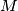$M$ is selected in the interior of the segment$AB$. The square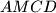$AMCD$ and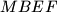$MBEF$ are constructed on the same side of$AB$, with segments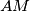$AM$ and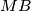$MB$ as their respective bases. The circles circumscribed about these squares, with centers$P$ and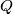$Q$, intersect at$M$ and also at another point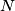$N$. Let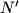$N'$ denote the point of intersection of the straight lines$AF$ and$BC$.

a) Prove that$N$ and$N'$ coincide;

b) Prove that the straight lines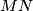$MN$ pass through a fixed point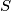$S$ independent of the choice of$M$;

c) Find the locus of the midpoints of the segments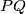$PQ$ as$M$ varies between$A$ and$B$.
Izvor: Međunarodna matematička olimpijada, shortlist 1959